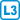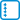## Eulerian sets

Let $$G = (V, E)$$ be a graph. Edge set $$E’ \subseteq E$$ is Eulerian if the graph $$(V, E’)$$ has all degrees even. (We do not the graph to be connected.)

• #### Variant

Prove that the set of all Eulerian sets of edges of a given graph is closed to a symmetric difference. Thus, prove that if $$E_1, E_2 \subseteq E$$ are Eulerian sets of edges, then, $$E_1 \Delta E_2$$ is also a Eulerian set of edges.

• #### Solution

If $$E_1$$ and $$E_2$$ are Eulerian, and $$v$$ is an arbitrary vertex, then the degree of $$v$$ in $$(V, E_1 \Delta E_2)$$ is equal to the sum of the degrees of the vertex $$v$$ in $$(V, E_1)$$ and $$(V, E_1)$$, but we have to subtract twice every edge with which $$v$$ is incident in the intersection of $$E_1 \cap E_2$$.

The sum resp. the difference of three even numbers is an even number, so $$E_1 \Delta E_2$$ is Eulerian.

• #### Variant

Prove that for every Eulerian set of edges $$E’$$ is $$G = (V, E’)$$ a union of edge disjoint cycles.

• #### Solution

We prove by induction according to the number of edges in $$E’$$.

If $$E ’$$ is empty, it is the empty union.

If it is non-empty, we choose any vertex of degree at least two and make any trail from it. Because there are at least two edges, this trail can be extended until some vertex $$v$$ on the trail repeats. The section of this trail between two occurrences of $$v$$ specifies the cycle $$C$$.

Because $$C \subseteq E’$$, we have that $$C \Delta E’= E’\setminus C$$ and therefore $$E’\setminus C$$ has fewer edges than $$E’$$. We know from the previous variant that $$E’\setminus C$$ is Eulerian and according to the inductive hypothesis it can be decomposed into disjoint union of cycles. By adding $$C$$ to this decomposition, we obtain the sought decomposition.

• #### Variant

Can you determine the number of all Eulerian sets of edges of a given graph?

• #### Hint

Choose any spanning forest (in the case of disconnected $$G$$ a union of spanning trees of its components) and call it skeleton. Each extra-skeletal edge creates a cycle together with the selected skeleton edges.

Show that these cycles are linearly independent while they generate the vector space of Eulerian sets. Their number determines the dimension of this space and the number of Eulerian sets can be derived from it.

• #### Solution

If $$e$$ is an extra-skeletal edge and $$C$$ is the corresponding cycle, then this edge cannot be eliminated by any linear combination of other cycles corresponding to extra-skeletal edges, simply because none of these cycles contain it. Therefore, the extra-skeletal cycle system is linearly independent.

Let $$K$$ be a fixed skeleton and $$E ’$$ be an arbitrary Eulerian set. We show that $$E’$$ can be expressed as a linear combination of cycles determined by the edges $$E’ \setminus K = \{e_1, \dots, e_k \}$$. Let $$C_i$$ be the cycle specified by the extra-skeletal edge $$e_i$$. The set $$R = E'\Delta C_1 \Delta \dots \Delta C_k$$ does not contain any extra-skeletal edge $$e_i$$, because these have exactly two occurrences in a symmetric difference: once in $$E'$$ and a second time in $$C_i$$.

So $$R \subseteq K$$ and also $$R$$ is Eulerian. Because each non-empty subgraph of a forest have to contain a leaf, i.e. a vertex of degree 1, $$R$$ must be empty, i.e. $$E’= C_1 \Delta \dots \Delta C_k$$. Thus, we have shown that cycles corresponding to extra-skeletal edges generate the space of Eulerian sets.

It remains to calculate that the union of skeletons of components $$G$$ has $$| V | -c$$ edges, where $$c$$ is the number of components of the graph $$G$$.

The number of Eulerian sets of a graph with $$c$$ connectivity components is $$2^{| E | - | V | + c}$$.